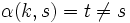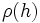# Tour:Equivalence of definitions of group action

PREVIOUS: Understanding the definition of a homomorphism| UP: Introduction five (beginners)| NEXT: Cayley's theorem
General instructions for the tour | Pedagogical notes for the tour | Pedagogical notes for this part
In an earlier page of this tour, you saw one definition of group action. There are actually two definitions of group action: the one you saw and another one in terms of homomorphism to a symmetric group. This page gives both definitions and proves their equivalence.
WHAT YOU NEED TO DO: Understand both definitions, and the proof of their equivalence.

## The definitions we have to prove as equivalent

### Definition as a map from a product

A group action of a group$G$ on a set$S$ is a map$\alpha:G \times S \to S$ satisfying:

1.$\alpha(gh,s) = \alpha(g,\alpha(h,s))$
2.$\alpha(e,s) = s$

Here$e$ denotes the identity element.

Further, the group action is termed faithful or effective if for every non-identity element$g \in G$, there exists$s \in S$ such that$\alpha(g,s) \ne s$.

### Definition as a homomorphism to a symmetric group

A group action of a group$G$ on a set$S$ is a homomorphism of groups$\rho:G \to \operatorname{Sym}(S)$ (where$\operatorname{Sym}(S)$ denotes the symmetric group on$S$).

Further, the action is termed faithful or effective if$\rho$ is injective.

## Proof of equivalence

### The first definition implies the second

Suppose we are given a map$\alpha:G \times S \to S$ satisfying the conditions:$\alpha(gh,s) = \alpha(g,\alpha(h,s))$

and:$\alpha(e,s) = s$

We now construct the map$\rho$. For every$g \in G$,$\rho(g)$ is defined as the map:$s \mapsto \alpha(g,s)$

Clearly,$\rho(g)$ is a function from$S$ to$S$, and$\rho(e)$ is the identity map (by the second assumption on$\alpha$. The fact that$\rho(g)$ is in$Sym(S)$ for every$g$ holds because:$\alpha(e,s) = \alpha(g^{-1}g,s) = \alpha(g^{-1}\alpha(g,s)) = \rho(g^{-1})(\rho(g)s)$

Hence$\rho(g^{-1})$ is a left inverse for$\rho(g)$, and a similar argument shows that it is a right inverse. Thus,$\rho(g)$ is an invertible map from$S$ to$S$, hence an element of$Sym(S)$.

Finally the fact that$\rho$ is a homomorphism follows from the first condition:$\rho(gh)(s) = \alpha(gh,s) = \alpha(g,\alpha(h,s)) = \alpha(g,\rho(h)s) = (\rho(g)\rho(h))(s)$

Further, suppose$\alpha$ is faithful. Consider any two distinct elements$g,h$ of$G$. Consider$k = hg^{-1}$. Since$g,h$ are distinct,$k$ is a non-identity element, so by faithfulness, there exists$s \in S$ such that$\alpha(k,s) = t \ne s$. Using the definition of group action, we obtain that$\alpha(h,\alpha(g^{-1},s)) = t$ while$\alpha(g,\alpha(g^{-1},s)) = s$. In particular,$\rho(h)$ and$\rho(g)$ are permutations that differ on$\alpha(g^{-1},s)$, hence are not equal. Thus, distinct elements of$G$ map to distinct permutations, and$\rho$ is thus injective.

### The second definition implies the first

Given a homomorphism$\rho:G \to \operatorname{Sym}(S)$, the map$\alpha$ is given by:$\alpha(g,s) = \rho(g)s$

Let us check that$\alpha$ satisfies both the specified conditions:

1.$\alpha(gh,s) = \rho(gh)s = (\rho(g)\rho(h))(s) = \rho(g)(\rho(h)s) = \rho(g)\alpha(h,s) = \alpha(g,\alpha(h,s))$
2.$\alpha(e,s) = \rho(e)s = s$

The latter is because a homomorphism of groups takes the identity element to the identity element.

Further, if$\rho$ is injective, then for any non-identity element$g \in G$,$\rho(g) \ne \rho(e)$, hence is not the identity permutation. Thus, there exists$s \in S$ such that$\rho(g)$ does not fix$s$. Thus yields$\alpha(g,s) \ne s$, as desired.